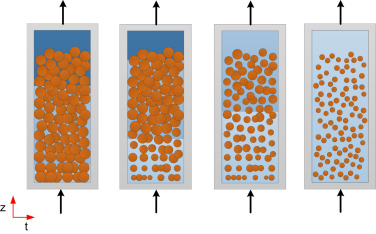Free software

EQUATION OF STATEEquations of State. Set of MATLAB programs for the calculation of phase equilibrium and other thermodynamic properties using different equations of state (classical cubic equations, cubic equations with excess Gibbs energy mixing rules, group contribution equations and SAFT equations). See more

HINT – HEAT INTEGRATION SOFTWAREThermogravimetric analysis modeling for biomass conversion. Excel/VBA software to model TGA curves from the fraction degradation viewpoint, i.e. hemicellulose, cellulose, lignin and extractables. See more  Paper  DownloadKinetic modelling for a biomass fracctionation process by hydrothermal treatment in subcritical water. Excel/VBA software to model TOC, pH and acetic acid curves at the outlet of a packed bed reactor. Paper Download

BEER DEALCOHOLIZATIONBeer dealcoholization via differential vacuum distillation can be simulated using Aspen HYSYS software. Here you can find a sample simulation file.

SUPERCRITICAL FLUID EXTRACTION (SFE)Supercritical Fluid Extraction from plants can be easily modelled and experimental data fit with this Excel application.   Download software and manual

Paper: Easy-understudying simulator for an extraction process of natural matrixes with supercritical CO2 in a packed bed column. The Journal of Supercritical Fluids.